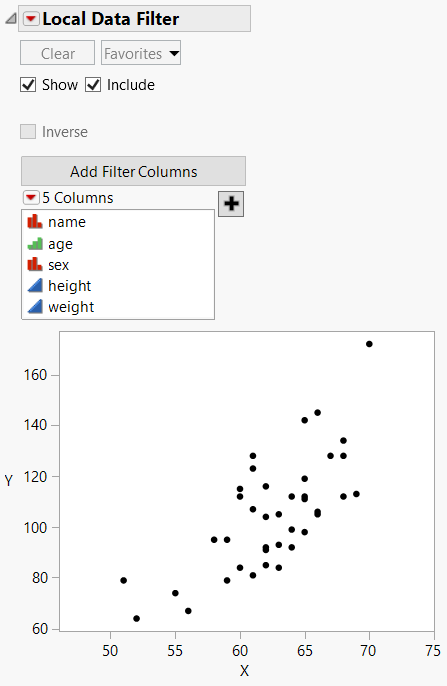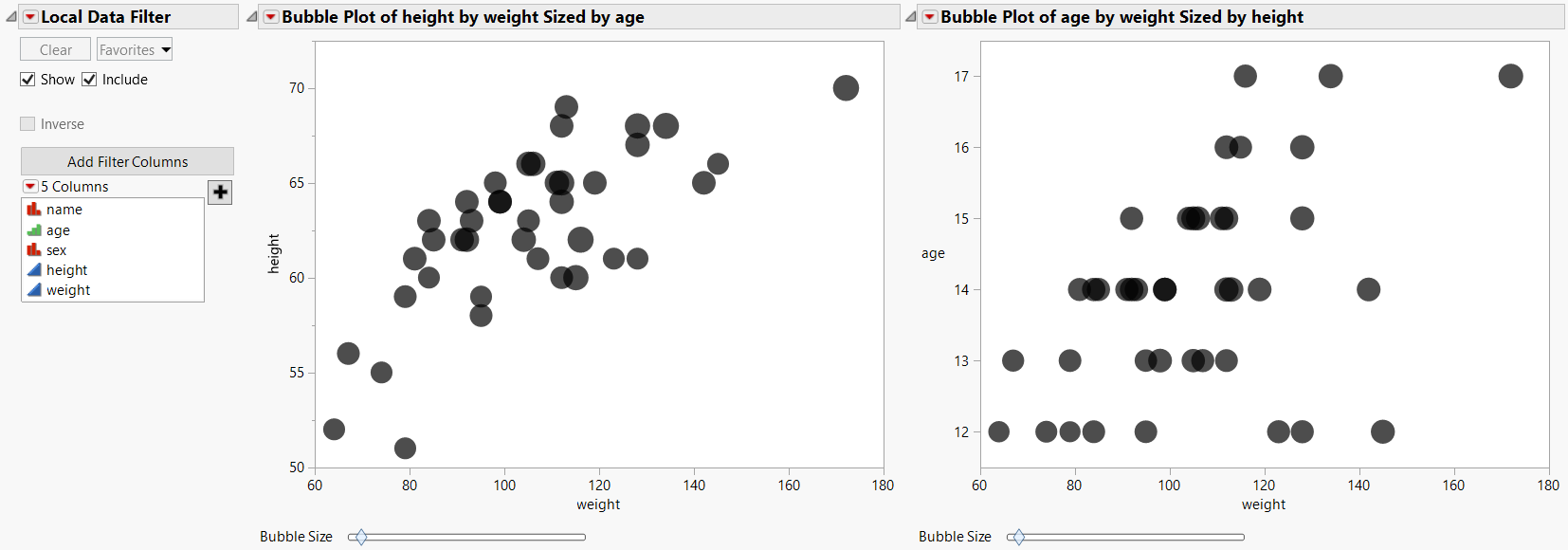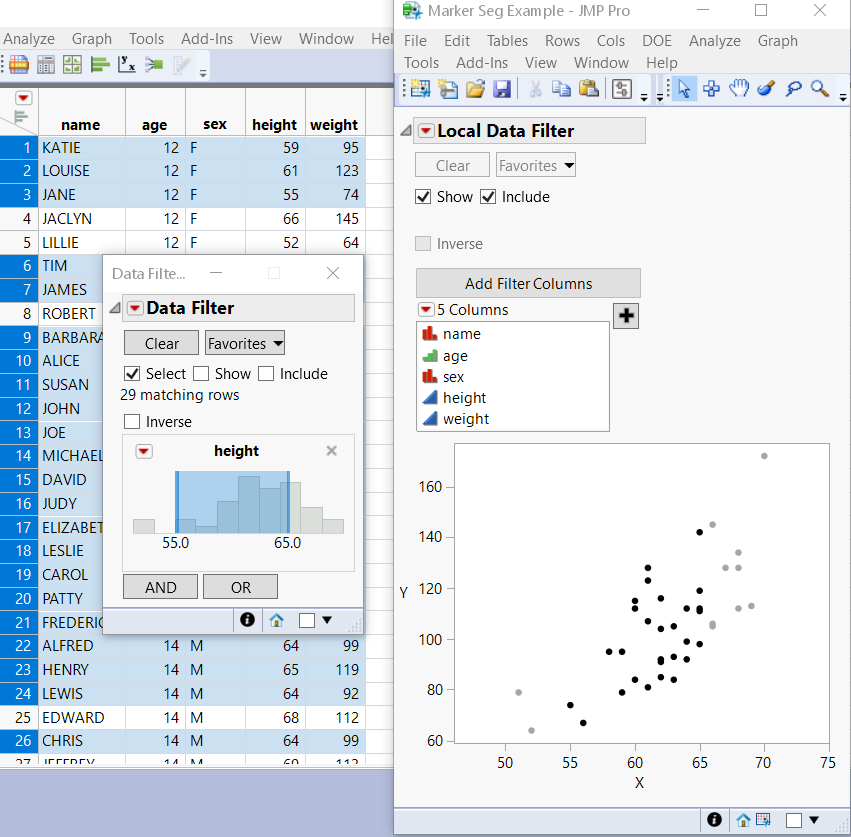Publication date: 05/24/2021

The Data Filter provides a variety of ways to identify subsets of data. Using Data Filter commands and options, you can select complex subsets of data, hide these subsets in plots, or exclude them from analyses.

Here is the basic syntax:

`dt << Data Filter(<Columns>, <Mode(...)>, <Add Filter(...)>, <Show Columns(), <Selector>, <Animation>, <Animate>, <Clear>, <Delete All>, <Delete>, <Filter Columns>, <Filter Column>, <Filter Group>, <Add Filter>, <Add>, <Match>, <Mode>, <Display>, <Where>, <Make Subset>, <Display>, <Show Histograms and Bars>)`

You can send an empty Data Filter message to a data table, and the initial Data Filter window appears, showing the Add Filter Columns panel that lists all the variables in the data table.

Mode takes three arguments, all of which are optional: Select(Boolean) Show(Boolean), Include(Boolean). These arguments turn on or off the corresponding options. The default value for Select is true (1). The default value for Show and Include is false (0).

Add Filter() adds rows and builds the WHERE clauses that describe a subset of the data table. Here is the basic syntax:

`Add Filter( Columns( col, ... ), Where( ... ), ... )`

To add columns to the data filter, list the columns names separated by commas. Note that this is not a list data structure.

You can define one or more WHERE clauses to specify the filtered columns:

`dt = Open( "\$SAMPLE_DATA/Big Class.jmp" );`
`df = dt << Data Filter(`
`	Mode( Show( 1 ) ),`
`	Add Filter(`
`		Columns( :age, :sex, :height ),`
`		Where( :age == {13, 14, 15} ),`
`		Where( :sex == "M" ),`
`		Where( :height >= 52 & :height <= 65 )`
`	)`
`);`

This script selects 13, 14, and 15-year-old males with a height greater than or equal to 52 and less than or equal to 65. In this filtered data, you can instead select the excluded ages by adding the Invert Selection message to the preceding script.

`df << ( Filter Column( :age ) << Invert Selection );`

In this example, ages other than 13, 14, and 15 in the filtered data are selected.

You can also use Add Filter() to select matching strings from columns with the Multiple Response property or Multiple Response modeling type.

`dt = Open( "\$SAMPLE_DATA/Consumer Preferences.jmp" );`
`df = dt << Data Filter(`
`	Location( {437, 194} ),`
`	Add Filter(`
`		Columns( :Brush Delimited ),`
`		Match None( Where( :Brush Delimited == {"Before Sleep", "Wake"} ) ),`
` `

/* Define the display options. "Brush Delimited" appears above

the list of values. Size is the size of the filter list. The

values appear next to check boxes. */

`		Display( :Brush Delimited, Size( 121, 70 ), Check Box Display )`
`		)`
`);`

This script selects rows with values in the Brush Delimited column that do not match either of the specified values ("Before Sleep", "Wake"). Other available scripting options include Match Any, Match All, Match Exactly, and Match Only. See Multiple Response in Using JMP for more information about the Multiple Response property and the Multiple Response modeling type.

You can also send messages to an existing Data Filter object:

`Clear(), Display( ... ), Animate(), Mode(), ...`

Clear takes no arguments and clears the data filter.

To prevent the data filter from appearing when the script is run, set Show Window to 0:

`obj  = ( dt << Data Filter( Show Window( 0 ) ) );`

To specify which values to exclude, use the != operator. The following example excludes ages 16 and 17 from the filtered values.

`dt = Open( "\$SAMPLE_DATA/Big Class.jmp" );`
`df = dt << Data Filter(`
`	Add Filter( Columns( :age, :sex ) ),`
`	Match( Columns( :age, :sex ),`
`		Where( :sex = "M" ), Where( :age != {16, 17} )`
`	)`
`);`

To omit the Data Filter outline and red triangle menu, specify No Outline Box.

`dt = Open( "\$SAMPLE_DATA/Cities.jmp" );`
`obj = dt << Data Filter(`
`	Add Filter( Columns( :Region, :POP ), Where( :Region == {"C", "N"} ) ),`
`	No Outline Box( 1 )`
`);`

### Add a Local Data Filter

A local data filter enables you to filter data in an individual report window without affecting other reports. When the local data filter is added to the platform, the reference to the data filter is returned. This provides an easy way to get access to the filter.

`dt = Open( "\$SAMPLE_DATA/Cities.jmp" );`
`biv = dt << Bivariate(`
`	Y( :x ),`
`	X( :y ),`
`	Automatic Recalc( 1 ),`
`	Fit Line( {Line Color( {213, 72, 87} )} )`
`);`
` `
`dfObj = biv << Local Data Filter(`
`// Include Show Histograms and Bars(0) to hide them in the filter.`

// Enable Include to include rows selected by the local data filter in

// calculations that are required to build graphs controlled by the local data

// filter. Deselected rows are excluded from calculations.

`	Location( {566, 47} ),`
`	Mode( Include( 1 ) )`
`);`
` `
`dfObj << Add Filter(`
`	Columns( :Region, :POP ),`
`	Where( :Region == {"C", "N"} )`
`);`

### Track Data Filter Changes

Make Filter Change Handler enables you to track changes to the data filter.

`dt = Open( "\$SAMPLE_DATA/Cities.jmp" );`
`dist = Distribution(`
`	Automatic Recalc( 1 ),`
`	Continuous Distribution( Column( :POP ) )`
`);`
`filter = dist << Local Data Filter(Add Filter( columns( :Region ) ));`
`f = Function( {a}, Print( a ) );`
`rs = filter << Make Filter Change Handler( f );`

### Define the Context of a Data Filter

A data filter lets you interactively select complex subsets of data, hide the subsets in plots, or exclude them from analyses. Your selections affect all analyses of the data table.

Another option is to filter data from specific platforms or display boxes. Create a local data filter inside the Data Filter Context Box() function. This defines the context as the current platform or display box rather than the data table.

The following example creates a local data filter for a Graph Box. See Figure 9.9 for the output.

`New Window( "Marker Seg Example",`
`	Data Filter Context Box(`
`		V List Box(`
`			dt << Data Filter( Local ),`
`			g = Graph Box(`
`				Frame Size( 300, 240 ),`
`				X Scale( Min( xx ) - 5, Max( xx ) + 5 ),`
`				Y Scale( Min( yy ) - 5, Max( yy ) + 5 ),`
`				Marker Seg( xx, yy, Row States( dt, rows ) )`
`			)`
`		)`
`	)`
`);`

Tip: The preceding script is part of a larger script that first builds the arrays required for a marker seg. To experiment with this script, open the Local Data Filter for Custom Graph.jsl sample script.

Figure 9.9 Local Data Filter and GraphA context box can also contain several graphs with one local filter. The filtering then applies to both graphs. The following script creates two bubble plots and one data filter in one context box. See Figure 9.10 for the output.

`dt = Open( "\$SAMPLE_DATA/Big Class.jmp" );`
`New Window( "Shared Local Filter",`
`	Data Filter Context Box(`
`		H List Box(`
`			dt << Data Filter( Local ),`
`			Platform(`
`				dt,`
`				Bubble Plot( X( :weight ), Y( :height ), Sizes( :age ) )`
`			),`
`			Platform(`
`				dt,`
`				Bubble Plot( X( :weight ), Y( :age ), Sizes( :height ) )`
`			)`
`		)`
`	)`
`);`

Tip: To experiment with this script, open the Local Data Filter Shared.jsl sample script.

Figure 9.10 Local Filter with Two Bubble PlotsData filters can be hierarchical. One script might generate a data filter and a local data filter. The outer data filter for the data table determines which data is available for the local data filter. To illustrate this point, the following script produces both types of data filters as shown in Figure 9.11.

Tip: The following script is part of a larger script that first builds the arrays required for a marker seg. See the Local Data Filter for Custom Graph.jsl sample script for code that builds those arrays.

`dt = Open( "\$SAMPLE_DATA/Big Class.jmp" );`
`...`
`New Window( "Marker Seg Example",`
`	Data Filter Context Box(`
`		V List Box(`
`			dt << Data Filter( Local ),`
`			g = Graph Box(`
`				Frame Size( 300, 240 ),`
`				X Scale( Min( xx ) - 5, Max( xx ) + 5 ),`
`				Y Scale( Min( yy ) - 5, Max( yy ) + 5 ),`
`				Marker Seg( xx, yy, Row States( dt, rows ) )`
`			)`
`		)`
`	)`
`);`

Heights greater than or equal to 55 and less than or equal to 65 are initially filtered. Then the user can work with the local data filter to filter columns on the graph.

Figure 9.11 Data Filter Hierarchy### Export a Report as Interactive HTML with a Live Data Filter

The preceding examples of adding a data filter enabled the Include mode. This mode includes rows selected by the local data filter in calculations that are required to build graphs controlled by the local data filter. Deselected rows are excluded from calculations.

For an Interactive HTML export, disable the Include mode, because Interactive HTML doesn’t exclude rows from calculations.

`dt = Open( "\$SAMPLE_DATA/Quality Control/Coating.jmp" );`
`dt << Graph Builder(`
`	Size( 532, 455 ),`
`	Show Control Panel( 0 ),`
`	Variables( X( :Sample ), Y( :Weight ) ),`
`	Elements( Points( X, Y, Legend( 3 ) ), Line( X, Y, Legend( 5 ) ) ),`
`	Local Data Filter( Mode( Include( 0 ) ), Add Filter( columns( :Weight ) ) )`
`);`
`Current Report() << Save Interactive HTML( "\$DESKTOP/example1.html" );`
`Web( "\$DESKTOP/example1.html" );`